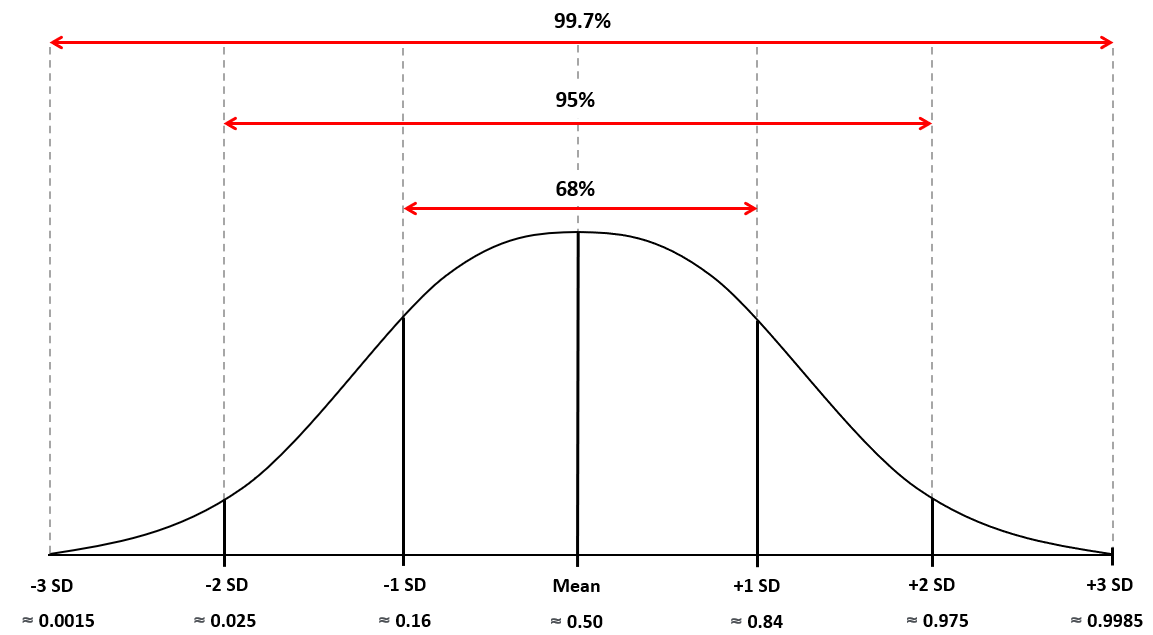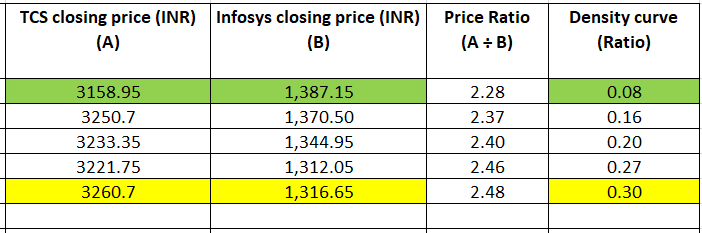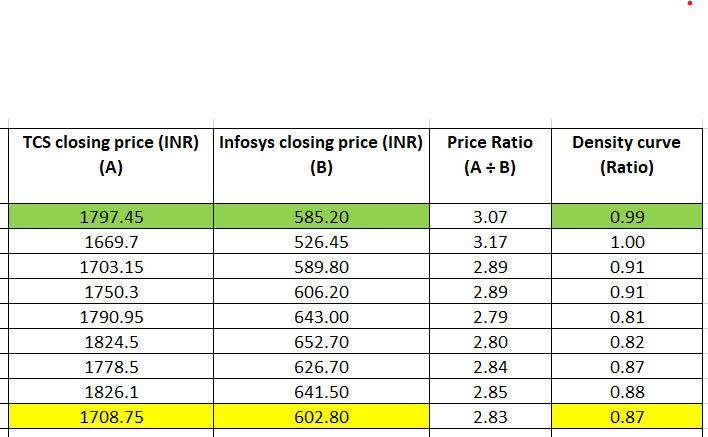# Applying the concepts to make the trade

5.0So far, you’ve stuck around and gotten the hang of a number of these statistical trade concepts. Let’s now apply trading logic to them and see how they can help you figure out what’s a good point to initiate a pair trade.

### Picking up where we left off: The density curve

Remember how we decided to take the ratio as the variable of choice for the purpose of this discussion? As you’ll recall, we calculated the mean for the price ratio for TCS and Infosys stocks (from 17 Feb, 2020 to 15 Feb, 2021) as 2.59.

So, if the price ratio for any given day is, say 2.28, you can expect it to rise back up to around 2.59 over time. Similarly, if the price ratio for another day is, say 3.07, you can expect it to fall back down around the mean region over time. But what is the probability of this mean reversion? As we saw in the previous chapter, the density curve reflects how likely it is for a variable to revert to the mean.

Take the following details for a given point during a trading session.

• Price ratio = 2.85
• Mean price ratio = 2.59
• Density curve = 0.89

So, you know the density curve in the given data set is 0.89. But how do you figure out the probability for this? Well, to decode this, we need to look at the bell curve again. Here’s the graph.A density curve of 0.0015 is around -3 SD from the mean. A density curve of 0.025 is around -2 SD from the mean. And so it goes. Remembering it may be hard, so here’s a table that you can quickly refer to in order to make sense of the density curve.

 Density curve Number of standard deviations to mean Probability of mean reversion 0.0015 -3 SD 99.7% 0.0250 -2 SD 95% 0.1600 -1 SD 68% 0.8400 +1 SD 68% 0.9750 +2 SD 95% 0.9985 +3 SD 99.7%

Now, back to the example we saw earlier. Recall the data points.

• Price ratio = 2.85
• Mean price ratio = 2.59
• Density curve = 0.89

Here, going by the table, a density curve of 0.89 falls between +1 SD and +2SD. But it is closer to +2 SD. So, it’s safe to say that the probability of a price ratio of 2.85 reverting to the mean price ratio of 2.59 is around 95%.

That’s how it’s done!

Depending on the probability of mean reversion and the direction of deviation, you will need to execute a long trade or a short trade. But wait up! If there are two assets in a pair trade, shouldn’t there be one long position and one short position in each pair trade? Yes, of course!

So, when we say ‘long trade,’ we essentially refer to the position you have to take in the asset that features in the numerator of your ratio. For example, we’ve taken the price ratio in our samples as:

 Stock price of TCS ÷ Stock price of Infosys

So, when we say take a long position, it means buy TCS, sell Infosys. That should clear this up.

Now, check out this quick guide to initiating a pair trade.

 Density curve range SD range Trade Target density curve Stop loss 0.0015 to 0.0250 -3 SD to -2 SD Long 0.025 or higher 0.0015 or lower 0.9750 to 0.9985 2 SD to 3 SD Short 0.975 or lower 0.9985 or higher

### Setting up a long trade

To set up a long trade, the trigger range for the density curve is from 0.0015 to 0.0250. Let’s take up some hypothetical data to understand what the trigger and the target scenario look like for a long trade.• In the row coloured green, the density curve is 0.08. Sure, it’s not in the range 0.0015 to 0.025, but it’s the lowest we have in our data set, and it’s quite close to the trigger range.
• Since this is a long trade, you will buy TCS at Rs. 3158.95 and sell Infosys at Rs. 1387.15.
• Recall from the table we saw earlier that the target density curve for a long trade is 0.025 or higher.
• The row coloured yellow meets our requirement.
• With a density curve of 0.30, that’s the target point.
• At this point, you will sell TCS at Rs. 3260.7 and buy Infosys at Rs. 1316.65.

So, what’s the net gain or loss from this trade? Let’s check it out.

 Trigger action Cash flow Target action Cash flow Net TCS Buy -3,158.95 Sell +3,260.7 101.75 Infosys Sell +1,387.15 Buy -1,316.65 70.50 172.25

So, this trade gives you a net profit of Rs. 172.25.

### Setting up a short trade

To set up a short trade, the trigger range for the density curve is from 0.9750 to 0.9985. Again, let’s take up some hypothetical data to understand what the trigger and the target scenario look like for a short trade.• In the row coloured green, the density curve is 0.99. This falls in the trigger range.
• Since this is a short trade, you will sell TCS at Rs. 1797.45 and buy Infosys at Rs. 585.20.
• Recall from the table we saw earlier that the target density curve for a short trade is  0.975 or lower.
• The row coloured yellow meets our requirement.
• With a density curve of 0.87, that’s the target point.
• At this point, you will buy TCS at Rs. 1708.75 and sell Infosys at Rs. 602.80.

So, what’s the net gain or loss from this trade? Let’s check it out.

 Trigger action Cash flow Target action Cash flow Net TCS Sell +1,797.45 Buy -1,708.75 88.70 Infosys Buy -585.20 Sell +602.80 17.60 106.30

So, this trade gives you a net profit of Rs. 106.30.

#### Wrapping up

Before we wrap up, here’s a quick reminder. Pair trading involves short selling. So, keep in mind that you can only execute pair trades in those market segments that support shorting. For the sake of simplicity, we may have used stock prices to discuss the trade concepts involved. However, in the spot market, you can only short sell if you’re executing intraday trades. So, if you want to pair trade in the spot market, you’ll need to execute the two trades within the trade day, and also close your positions in that time frame.

Alternatively, you can execute pair trading strategies in the futures market. In fact, that’s the most common way pair trading is done.

#### A quick recap

• The density curve can tell you when to initiate a pair trade.
• Generally, it’s ideal to consider trigger points where the density curve falls in between the 2 SD and 3SD range, or the -3 SD and -2 SD range.
• When the density curve is between -2 SD and -3SD, you initiate a long trade.
• When the density curve is between +2 SD and +3SD, you initiate a short trade.
• A long trade, in the context of pair trading, means you buy the numerator stock and sell the denominator stock (with regard to the price ratio).
• A short trade, in the context of pair trading, means you sell the numerator stock and buy the denominator stock (with regard to the price ratio).